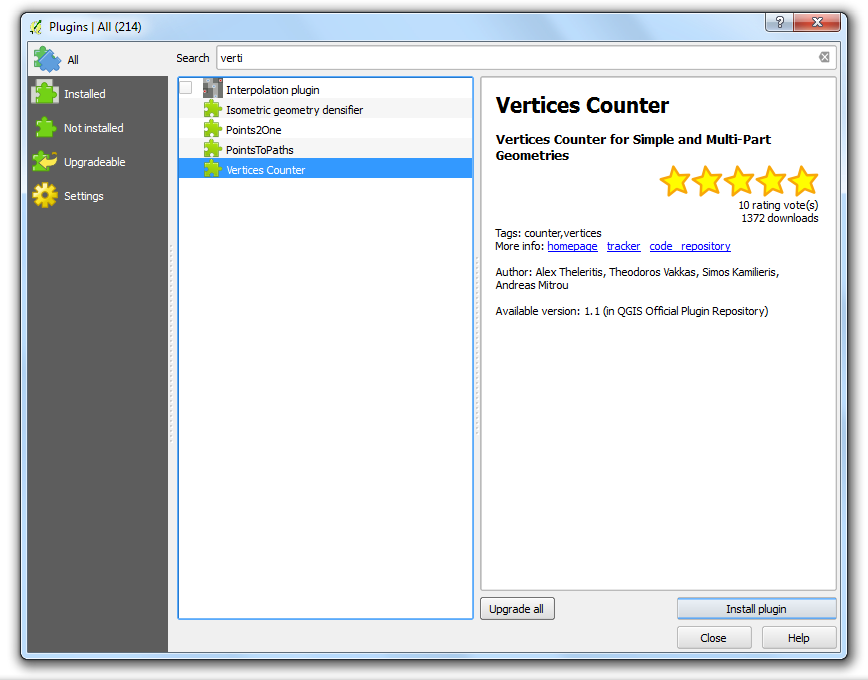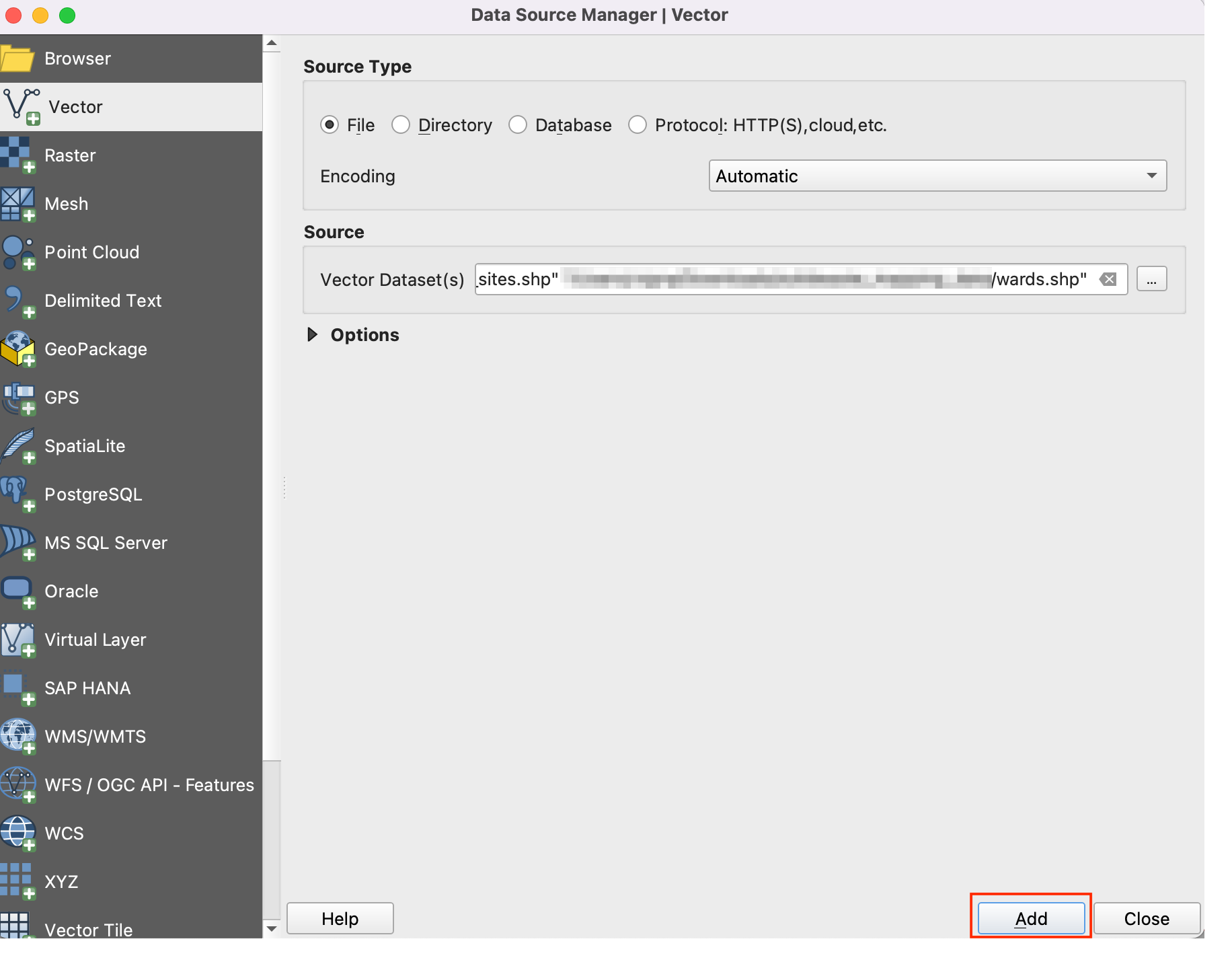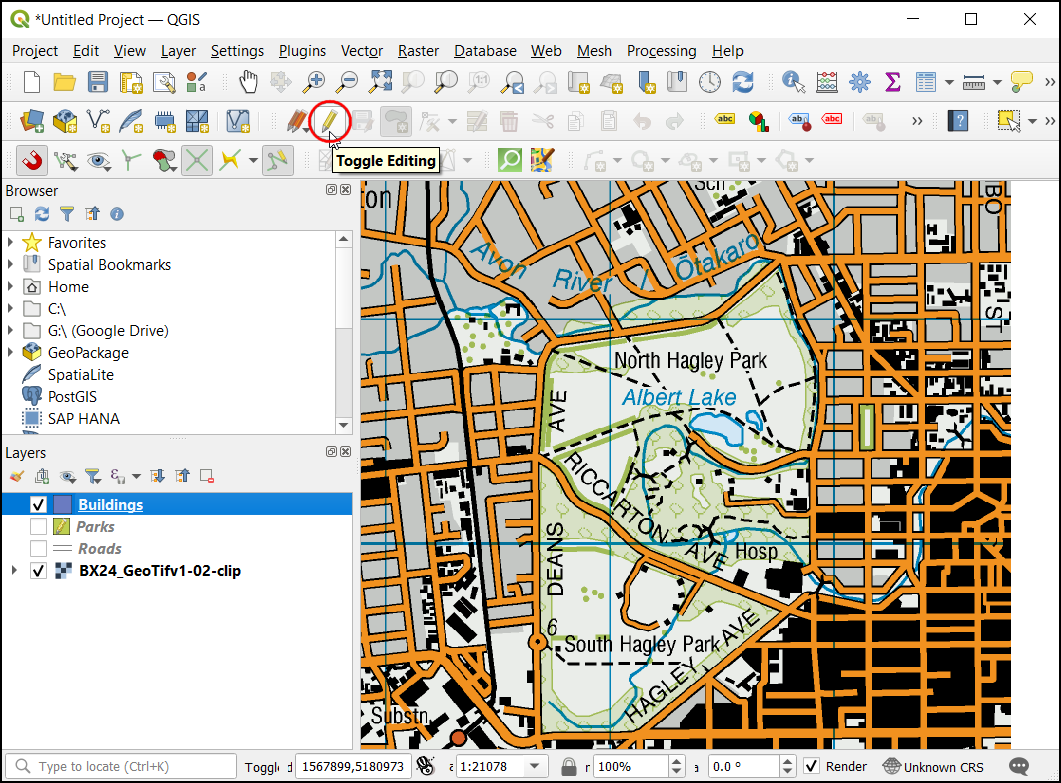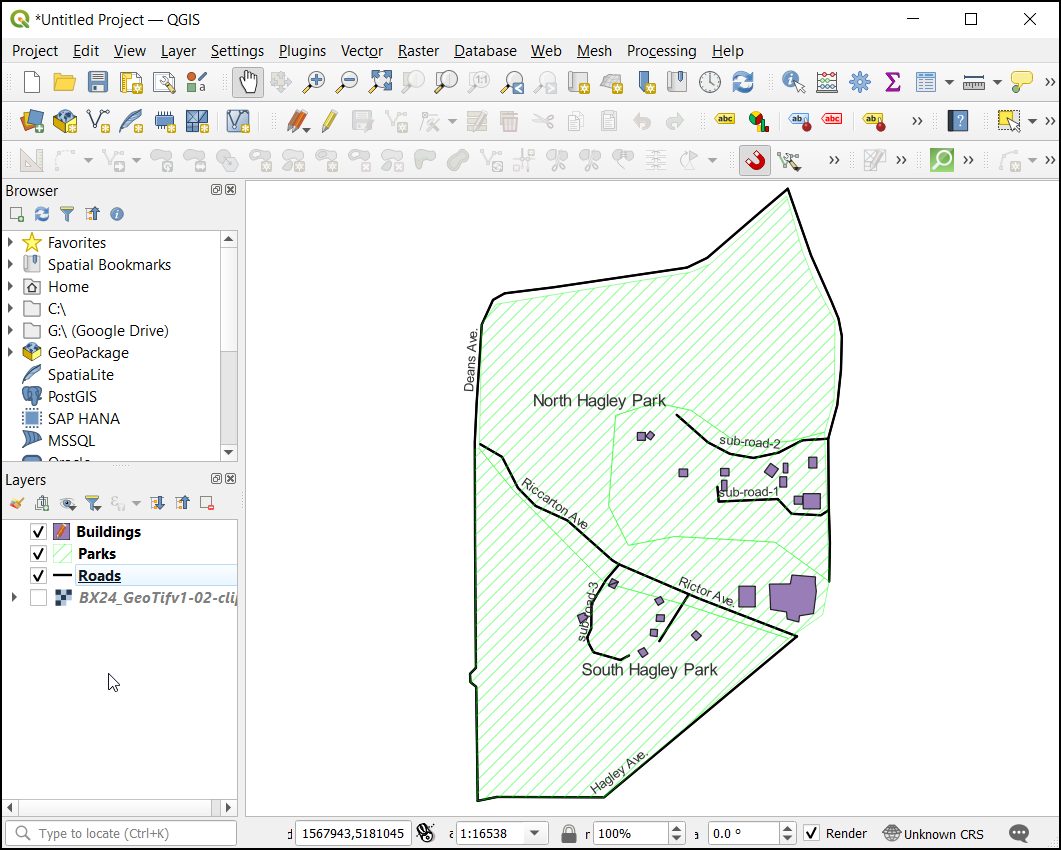# Realización de uniones de tabla (PyQGIS)¶

This tutorial shows how to use Python scripting in QGIS (PyQGIS) to perform a table join and apply a graduated style to the resulting layer. This tutorial replicates the steps of the Realización de uniones de tabla tutorial using only python scripting.

Please refer to Realización de uniones de tabla tutorial for the overview.

### Other skills you will learn¶

• Using QgsGraduatedSymbolRendererV2 to apply a graduated style to a vector layer.

## Obtener los datos¶

tl_2013_06_tract.zip

ca_tracts_pop.csv

ca_tracts_pop.csvt

Data Source [TIGER] [USCENSUS]

## Procedmiento¶

You can type the following commands in the Python Console or the built-in Editor in QGIS.

1. Load the shapefile. The Census Tracts file is a zip file containing the shapefile. While we can unzip it and load the shapefile, The OGR provider has the ability to load the zip file directly via a Virtual Filesystem. Adding `/vsizip/` in the path, we can access the shapefile contained in the zip archive.

Nota

The zip_uri would begin with `/vsizip//` on Linux and Mac systems. (Note the extra /)

```zip_uri = '/vsizip/C:/Users/Ujaval/Downloads/tl_2013_06_tract.zip'
shp =  QgsVectorLayer(zip_uri, 'tl_2013_06_tract', 'ogr')
```1. Load the CSV file. As the CSV file doesn’t contain any spatial data, we load it as a table using the `delimitedtext` provider.
```csv_uri = 'file:///C:/Users/Ujaval/Downloads/ca_tracts_pop.csv?delimiter=,'
csv = QgsVectorLayer(csv_uri, 'ca_tracts_pop', 'delimitedtext')
```1. Create the table join. Table joins in QGIS are performed using `QgsVectorJoinInfo` object. We need to specify the `GEO.id2` field from the CSV layer as the Join Field and the `GEOID` field from the shapefile layer as the Target Field. Once you run the following code, the shapefile layer will have additional attributes joined from the csv layer.

Nota

A common pitfall when using QgsVectorJoinInfo is that both the layers must be loaded in the QgsMapLayerRegistry - otherwise the join would not work.

```shpField='GEOID'
csvField='GEO.id2'
joinObject = QgsVectorJoinInfo()
joinObject.joinLayerId = csv.id()
joinObject.joinFieldName = csvField
joinObject.targetFieldName = shpField
joinObject.memoryCache = True
```1. An easier - and preferred way of accomplishing the same thing is via the Processing Framework. You can call the algorithm `qgis:joinattributestable` and create a joined layer.

Nota

We are using the `processing.runandload()` method to execute the algorithm instead of the more common `processing.runalg()`. Since we want to load the resulting joined layer in QGIS, `processing.runandload()` is a better choice.

```import processing
shpField='GEOID'
csvField='GEO.id2'
result = processing.runandload('qgis:joinattributestable', shp, csv, shpField, csvField, None)
```1. We will stick with the original join using `QgsVectorJoinInfo` for the remainder of the tutorial. Now it is time to apply a graduated style to the joined layer. The population field name in the joined layer is `ca_tracts_pop_D001`. We will apply a graduated renderer using the `QgsGraduatedSymbolRendererV2` class in the `Quantile` mode. Refer to Realización de uniones de tabla for the colors and ranges that we need to use.
```from PyQt4 import QtGui

myColumn = 'ca_tracts_pop_D001 '
myRangeList = []
myOpacity = 1

ranges = []

myMin1 = 0.0
myMax1 = 3157.2
myLabel1 = 'Group 1'
myColor1 = QtGui.QColor('#f7fbff')
ranges.append((myMin1, myMax1, myLabel1, myColor1))

myMin2 = 3157.2
myMax2 = 4019.0
myLabel2 = 'Group 2'
myColor2 = QtGui.QColor('#c7dcef')
ranges.append((myMin2, myMax2, myLabel2, myColor2))

myMin3 = 4019.0
myMax3 = 4865.8
myLabel3 = 'Group 3'
myColor3 = QtGui.QColor('#72b2d7')
ranges.append((myMin3, myMax3, myLabel3, myColor3))

myMin4 = 4865.8
myMax4 = 5996.4
myLabel4 = 'Group 4'
myColor4 = QtGui.QColor('#2878b8')
ranges.append((myMin4, myMax4, myLabel4, myColor4))

myMin5 = 5996.4
myMax5 = 37452.0
myLabel5 = 'Group 5'
myColor5 = QtGui.QColor('#08306b')
ranges.append((myMin5, myMax5, myLabel5, myColor5))

for myMin, myMax, myLabel, myColor in ranges:
mySymbol = QgsSymbolV2.defaultSymbol(shp.geometryType())
mySymbol.setColor(myColor)
mySymbol.setAlpha(myOpacity)
myRange = QgsRendererRangeV2(myMin, myMax, mySymbol, myLabel)
myRangeList.append(myRange)

myRenderer.setClassAttribute(myColumn)

shp.setRendererV2(myRenderer)
```1. Typing the code in the Python Console is useful for small tasks, but it is far easier to use the built-in Editor. You can copy the entire script in the Editor and click Run. As the script finishes, you would have created a table join and styled the resulting layer without any manual steps.Below is the full `join_attributes.py` file as a reference.

```from PyQt4 import QtGui
shp =  QgsVectorLayer(zip_uri, 'tl_2013_06_tract', 'ogr')

csv = QgsVectorLayer(csv_uri, "ca_tracts_pop", "delimitedtext")

shpField='GEOID'
csvField='GEO.id2'
joinObject = QgsVectorJoinInfo()
joinObject.joinLayerId = csv.id()
joinObject.joinFieldName = csvField
joinObject.targetFieldName = shpField
joinObject.memoryCache = True

myColumn = 'ca_tracts_pop_D001 '
myRangeList = []
myOpacity = 1

ranges = []

myMin1 = 0.0
myMax1 = 3157.2
myLabel1 = 'Group 1'
myColor1 = QtGui.QColor('#f7fbff')
ranges.append((myMin1, myMax1, myLabel1, myColor1))

myMin2 = 3157.2
myMax2 = 4019.0
myLabel2 = 'Group 2'
myColor2 = QtGui.QColor('#c7dcef')
ranges.append((myMin2, myMax2, myLabel2, myColor2))

myMin3 = 4019.0
myMax3 = 4865.8
myLabel3 = 'Group 3'
myColor3 = QtGui.QColor('#72b2d7')
ranges.append((myMin3, myMax3, myLabel3, myColor3))

myMin4 = 4865.8
myMax4 = 5996.4
myLabel4 = 'Group 4'
myColor4 = QtGui.QColor('#2878b8')
ranges.append((myMin4, myMax4, myLabel4, myColor4))

myMin5 = 5996.4
myMax5 = 37452.0
myLabel5 = 'Group 5'
myColor5 = QtGui.QColor('#08306b')
ranges.append((myMin5, myMax5, myLabel5, myColor5))

for myMin, myMax, myLabel, myColor in ranges:
mySymbol = QgsSymbolV2.defaultSymbol(shp.geometryType())
mySymbol.setColor(myColor)
mySymbol.setAlpha(myOpacity)
myRange = QgsRendererRangeV2(myMin, myMax, mySymbol, myLabel)
myRangeList.append(myRange)